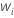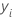# Methods and formulas for the response information in Fit Model and Discover Key Predictors with TreeNet® Classification

###### Note

The values in the response information table are for the response variable and do not depend on the validation method. For an unweighted analysis the response information table includes the counts of events and non-events. The table also shows the percentages of each class:

For a weighted analysis, the table displays information about 2 types of weights in the response information table: class weights and case weights.

## Class weights

For an analysis with class weights, Minitab provides the option to weight the classes to ensure equal sample sizes across classes. For this type of analysis, the most frequent class has a weight of 1. The following calculation determines the weights for the remaining classes for a binary response:
The following calculation generalizes the class weights for a multinomial response:

## Case weights

For an analysis with case weights, the weights are in a column that has the same number of rows as cases in the data. The weighted count for a class has the following formula:

## Notation

TermDescription
Nsample sizeweight for the ith case in the dataindicator variable that is 1 for the class of interest and 0 otherwise
By using this site you agree to the use of cookies for analytics and personalized content.  Read our policy